Introduction to Thermochemistry & Second Law of Thermodynamics

# Introduction to Thermochemistry & Second Law of Thermodynamics Notes | Study Physical Chemistry - Chemistry

## Document Description: Introduction to Thermochemistry & Second Law of Thermodynamics for Chemistry 2022 is part of Physical Chemistry preparation. The notes and questions for Introduction to Thermochemistry & Second Law of Thermodynamics have been prepared according to the Chemistry exam syllabus. Information about Introduction to Thermochemistry & Second Law of Thermodynamics covers topics like and Introduction to Thermochemistry & Second Law of Thermodynamics Example, for Chemistry 2022 Exam. Find important definitions, questions, notes, meanings, examples, exercises and tests below for Introduction to Thermochemistry & Second Law of Thermodynamics.

Introduction of Introduction to Thermochemistry & Second Law of Thermodynamics in English is available as part of our Physical Chemistry for Chemistry & Introduction to Thermochemistry & Second Law of Thermodynamics in Hindi for Physical Chemistry course. Download more important topics related with notes, lectures and mock test series for Chemistry Exam by signing up for free. Chemistry: Introduction to Thermochemistry & Second Law of Thermodynamics Notes | Study Physical Chemistry - Chemistry
 1 Crore+ students have signed up on EduRev. Have you?

THERMOCHEMISTRY

Change in Internal energy in a chemical reaction:

From first law dq = dU – w

If a chemical reaction takes place at constant temperature and constant volume. Then W = 0

Hence ΔU = qv = heat exchange at constant volume

Suppose V& UP be the internal energy of reactant and products.
then, ΔU = UP - UR
= qv = Heat or enthalpy of reaction at constant volume

Change in Enthalpy a chemical reaction: Let qp be the heat exchange in the reaction at constant pressure. Then
ΔH = qP
If H& HP be the enthalpy of reactant and product then

ΔH = HP - HR
= qp = heat or enthalpy o f reaction at constant pressure

Relation between Enthalpy of reaction at constant volume and a constant pressure:

We know that
ΔH = qP & ΔU = qV
then qP = qV + PΔV
Forn moles of an ideal gas
PV = nRT

Let n1 & n2 be the number of moles of gaseous reactants & products.
then                  Δn = n2 - n1 = increase in the number of gaseous moles
Then corresponding increase in volume ΔV is given by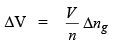Hence,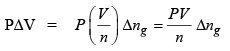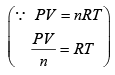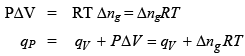Then           qP = qV + Δng RT

Variation of enthalpy of reaction with temperature i.e., Kirchhoff equation.
The enthalpy change for the reaction
aA + bB → cC + dD

is given by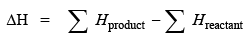The temperature dependence of enthalpy at constant pressure is:
then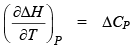or d(DH) = DCPdT ...(1) The temperature dependence of enthalpy of constant volume is: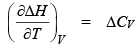or d(ΔU) = ΔCVdT ...(2)
Integrating equation (1) & (2) we get

or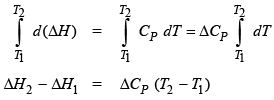Similarly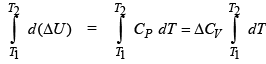or ΔU2 - ΔU1 = ΔCV (T2 - T1 )
Above equations are known as Kirchhoff equations.
Problem.  Calculate ΔHo373 for the reaction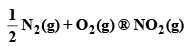ΔH298 K = -33.18 KJ mol-1
Given, CP (NO2, g) = 37.20 J K-1 mol-1
CP (N2, g) = 29.13 JK-1 mol-1
CP (O2, g) = 29.36 JK-1 mol-1s
Sol.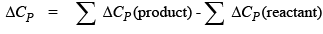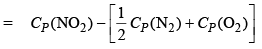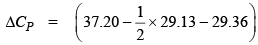= - 6.73 x 10-3JK mol-1
Now,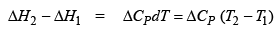ΔH0373 K - ΔH0 298 K = ΔCP (373 - 298)

•  ΔH0 373 K = [-33.18 + (-6.73 × 10-3) (373 - 298)] KJ mol-1
• ΔH0 373 K = -33.68 KJ mol-1

The second law of Thermodynamics: The second law of thermodynamics identifies a new state function called the entropy which provides a criterion for identifying the equilibrium state of a system.
Entropy of the universe (system + surrounding) increases for irreversible process whereas it remains constant for reversible process.

Carnot cycle: Consider the system is contained in a frictionless piston and cylinder arrangement. We also use two thermal reservoirs one at higher temperature T2 and other one at a lower temperature T1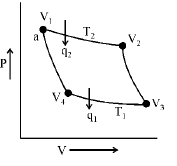Four successive operations as given below:
(1)  The isothermal reversible expansion from volume V1 to volume L at the higher temperature T2 .
Since dT = 0, therefore, ΔU1 = 0 According to first law of thermodynamics, we have q2 = 2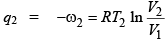(2)  The adiabatic reversible expansion from volume V2 to volume V3.
Temperature of the system after expansion is T1. Since for adiabatic process q = 0, therefore, it follows that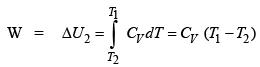(3)  The isothermal reversible compression from V3 to V4 at temperature T1.
Here             ΔU3 = 0
and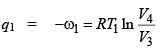(4)  Adiabatic reversible compression from volume V4 to V1.
Temperature of the system returns T2. Therefore,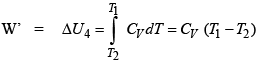Newt work involved in the cyclic process is:

Wtotal = W2 + W + W1 + W’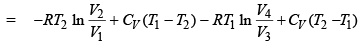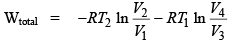Relationship between V1, V2, V3 & V4: Second and fourth process are adiabatic expansion and compression process then from poisson equation we get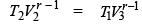(for second process)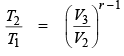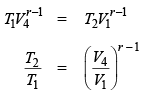From equation (A) & (B) we get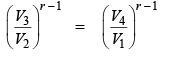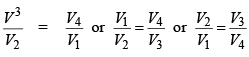then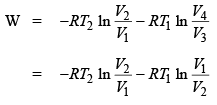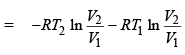Wtotal =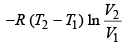Efficiency of Carnot cycle: It is denoted by η.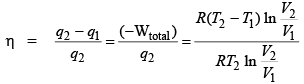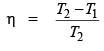Thus, the efficiency of a heat engine operating in a Carnot cycle depends only on the two temperatures and primarily, on the difference of the two temperatures between which the engine operators.
The difference is greater, the greater the efficiency.

Problem. An engine operator in a Carnot cycle absorb 3.347 kJ at 400°C, how much work is done on the engine per cycle and how much heat is involved at 100°C in each cycle?
Sol.
The efficiency of Carnot cycle is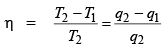Thus,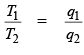the heat involved is:
q1 = 1.855 kJ                                             (heat is negative sign)
q1 = –1.855 kJ
and work done is :
W = q– q1 = –3.347 + 1.855 = –1.492 kJ

Coefficient of performance: The coefficient of performance of a refrigerator is defined as the ratio of heat transferred from a lower temperature to a higher temperature to the work done on the machine to cause this removal i.e.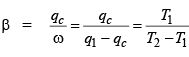Relation between η & β :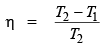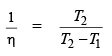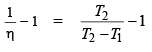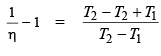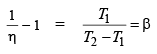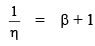i.e.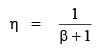The document Introduction to Thermochemistry & Second Law of Thermodynamics Notes | Study Physical Chemistry - Chemistry is a part of the Chemistry Course Physical Chemistry.
All you need of Chemistry at this link: Chemistry

## Physical Chemistry

67 videos|106 docs|31 tests
 Use Code STAYHOME200 and get INR 200 additional OFF

## Physical Chemistry

67 videos|106 docs|31 tests

Track your progress, build streaks, highlight & save important lessons and more!

,

,

,

,

,

,

,

,

,

,

,

,

,

,

,

,

,

,

,

,

,

;